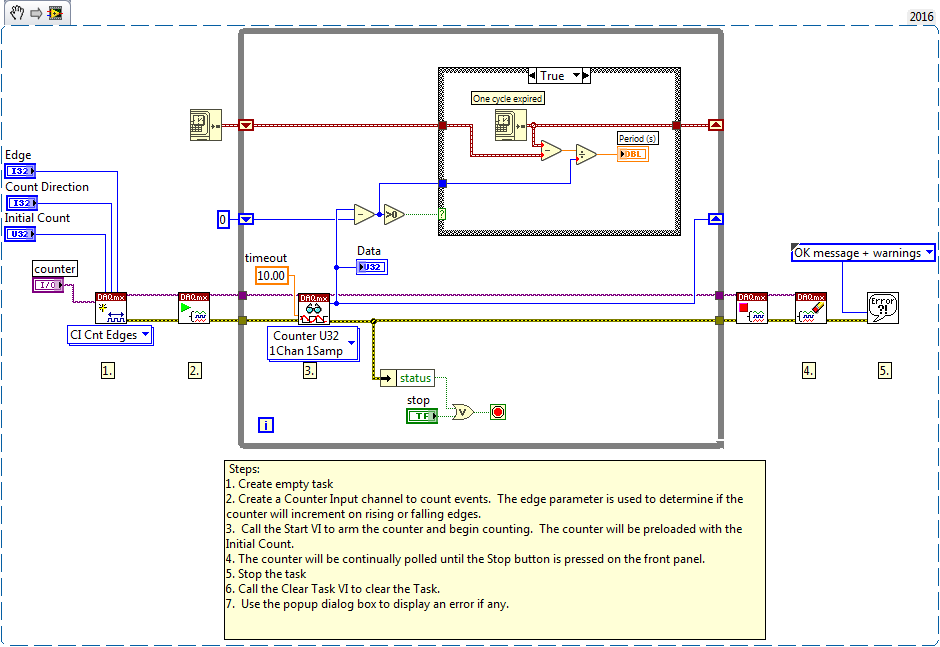Using the USB-6008/6009 To Measure Periods

Publish Date: Feb 21, 2018 | 5 Ratings | 3.60 out of 5 | Print | Submit your review

Overview

This document describes the method for measuring the period of a signal using the USB-6008 or USB-6009 device counter.

1. Introduction

Since the USB-6008/6009 has a 32 bit counter without a gate, the device can only count edges. This means that the device cannot perform period or frequency measurement directly in hardware.

You can, however, use edge counting and measure the time period between two events. To do this, take the time difference divided by the number of counted edges to get the approximate period of the signal. A shift register is used to ensure a change of counter value has occurred.

[time of previous edge - time of current edge] / [current edge count - previous cycle edge count]

The attached example calculates a running average for the period by dividing the total edges counted by the total time elapsed. This provides accurate period measurements for both low and high frequencies, however it cannot measure a dynamic period.

Note: The DAQmx Base version of this example is intended for Macintosh and Linux users. The DAQmx version of this example is intended for Windows users.